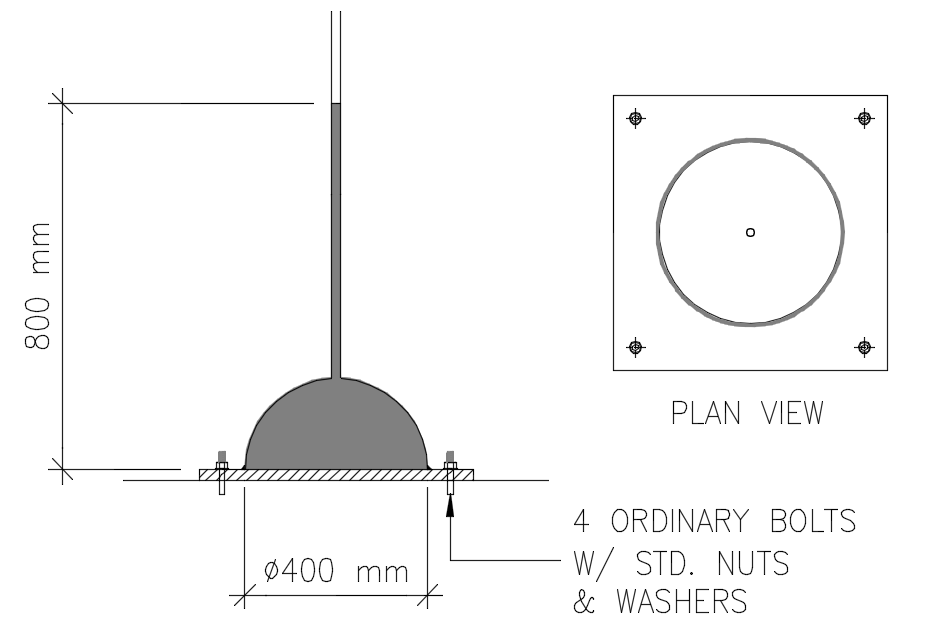# Diameter of bolts

2 posts / 0 new
Engr JaydeeDiameter of boltsA hollow hemispherical shell is fixed to the ground by using four ordinary bolts and a steel base plate. A small tube is installed on top of it. To the tube, water is poured up to a height of 800 mm from the bottom of the shell. See figure above.

1. Calculate the diameter of the bolts (in mm) that can safely carry an allowable bolt axial stress of 1 MPa.
2. Calculate the diameter of the bolts (in mm) that can safely carry an allowable bolt shear stress of 500 kPa.
3. Based on your answers in #1 and #2, suggest the best bolt diameter for the setup. Commercial sizes are 12 mm, 16 mm, 20 mm, 25 mm, 28 mm, 32 mm and 36 mm.

Jhun VertTry ko lang sir.

The total hydrostatic force is acting upward:
$F = \gamma V = 9810 \left[ \dfrac{\pi}{4}(0.4^2)(0.8) - \dfrac{1}{2} \cdot \dfrac{4}{3}\pi (0.2^3) \right]$

$F = 821.84 \text{ N}$

Tensile force carried by each bolt
$T = \frac{1}{4}F = 205.46 \text{ N}$

Diameter of bolt based on axial stress
$\sigma = \dfrac{T}{A}$

$1 = \dfrac{205.46}{\frac{1}{4}\pi d^2}$

$d = 16.17 \text{ mm}$

Not sure kung tama pagka analyze ko at hindi ko pa naisip paano kukunin ang due sa shear. Naghahanap kasi ako ng thickness the bolt-head or thickness ng nuts. Ang naisip ko lang kasi na shear ay yung shear failure sa thread or shear failure ng head. I can't imagine pa how to solve with shear na parallel sa area ng bolt ang force.

Parang nakita ko na 'to na problem sa file ko long ago, triny ko pagkalkal pero di ko nahanap, hehehe.

• Mathematics inside the configured delimiters is rendered by MathJax. The default math delimiters are $$...$$ and $...$ for displayed mathematics, and $...$ and $...$ for in-line mathematics.